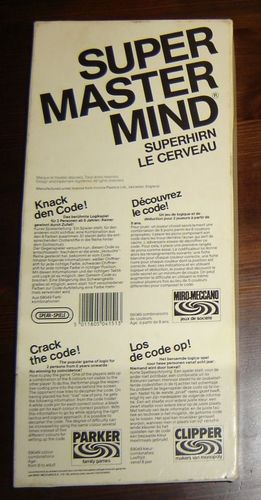# How to create a Super Mastermind AI

The Super MasterMind is a 70s fancy board game (it’s an advanced version of the classic MasterMind), where a player has to find turn by turn the hidden combination of 5 colored pins chosen by the opponent.

At each turn, the player proposes a combination of colored pins and the opponent tells him how many pins colors are well placed, and how many are not. Based on those indications, the player tries a new combination. The goal is to find the right combination with the least number of guesses.## Problem

Think about how to make a simple AI (the word is maybe a bit promotional) to guess the combination. It’s a good exercise for a beginner in programming.

A good warmup could be to code the one or two functions which take the secret combination and an attempt of the player in input, and return the number of well-placed and not well placed pins.

## Solution

Ok, so the idea is pretty straightforward. Start with the set of all possible combinations. At each turn of the game, just remove all combinations that don’t respect the constraint of the given information (of well-placed and not well-placed pins) of the opponent. Pick randomly (or not) a combination of pins in the remaining set, loop on these instructions until the set contained only one remaining combination.

I let you a C++ solution I wrote while I was a teenager (don’t judge too hard the fancy AINSI colors) - That was while a raining day on l’Ile d’Yeux! I rewrote it in Rust a few years ago: /mastermind

``````#include <iostream>
#include <string>
#include <vector>

#define P 5 // number of pawns
#define C 8 // number of colors

#define MAX 32768 // C^P

std::string print_pawns(int p) {
std::string str = "[ ";
for (int i = 0; i < P; ++i) {
switch (p % C) {
case 0:
str += " Bla";
break;
case 1:
str += " Whi";
break;
case 2:
str += " Red";
break;
case 3:
str += " Gre";
break;
case 4:
str += " Blu";
break;
case 5:
str += " Yel";
break;
case 6:
str += " Ora";
break;
case 7:
str += " Bro";
break;
}
p /= C;
}
return str;
}

int get_number_of_well_placed_pawn(int a, int b) {
int c = 0;
for (int i = 0; i < P; ++i) {
if (a % C == b % C) {
++c;
}
a /= C;
b /= C;
}
return c;
}

int get_number_of_good_colors(int a, int b) {
std::vector<bool> x(C, false);
std::vector<bool> y(C, false);
for (int i = 0; i < P; ++i) {
x[a % C] = true;
y[b % C] = true;
a /= C;
b /= C;
}
int c = 0;
for (int i = 0; i < C; ++i) {
if (x[i] && y[i]) {
++c;
}
}
return c;
}

int get_number_of_colors(int p) {
std::vector<bool> x(C, false);
for (int i = 0; i < P; ++i) {
x[p % C] = true;
p /= C;
}
int c = 0;
for (int i = 0; i < C; ++i) {
if (x[i]) {
++c;
}
}
return c;
}

int main(void) {
int p = 0;
std::vector<bool> vec(MAX, true);
for (int i = 0; i < MAX; ++i) {
if (get_number_of_colors(i) != P) {
vec[i] = false;
}
}
for (int turn = 1; true; ++turn) {
int n = 0;
for (int i = 0; i < MAX; ++i) {
if (vec[i]) {
++n;
p = i;
}
}
if (!n) {
break;
}
std::cout << "\x1b[33m.. sequences:\x1b[0m " << n << std::endl;
std::cout << "\x1b[33m.. turn " << turn;
std::cout << ": \x1b[0m" << print_pawns(p) << std::endl;
int b = 0, w = 0;
std::cout << "\x1b[34m>> black:\x1b[0m ";
std::cin >> b;
std::cout << "\x1b[34m>> white:\x1b[0m ";
std::cin >> w;
if (b < 0 || 5 < b || w < 0 || 5 < w) {
std::cout << "\x1b[31m.. error:\x1b[0m wrong input" << std::endl;
break;
}
if (b == P) {
std::cout << "\x1b[32m.. success:\x1b[0m I win!" << std::endl;
break;
}
vec[p] = false;
for (int i = 0; i < MAX; ++i) {
if (vec[i] && (get_number_of_well_placed_pawn(p, i) != b
|| get_number_of_good_colors(p, i) != b + w)) {
vec[i] = false;
}
}
}
return 0;
}
``````

## Go further

A good combinatorial / statistics question could be: What is the average number of steps to find the solution for a combination of N pins, which M available colors?

Donald Knuth gives the answer and offers a way to improve our simple AI, more information can be found on https://en.wikipedia.org/wiki/Mastermind_(board_game)#Algorithms!